Home > CC4 > Chapter A > Lesson A.1.2 > ProblemA-22

A-22.

If f(x) = 2x + 3, evaluate each expression below. Homework Help ✎

1. f(−5)

2. f(−)

3. f(4)

4. f(−2)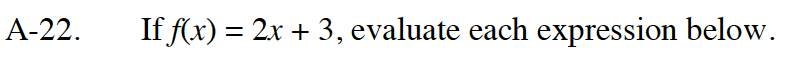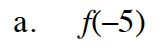f(−5) = 2(−5) + 3

f(−5) = −10 + 3

f(−5) = −7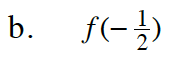Follow the steps in part (a).

2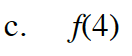Follow the steps in part (a).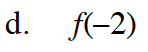Follow the steps in part (a).